# 射频 - 谐振电路 - 无损组件的共振

## 无损组件的共振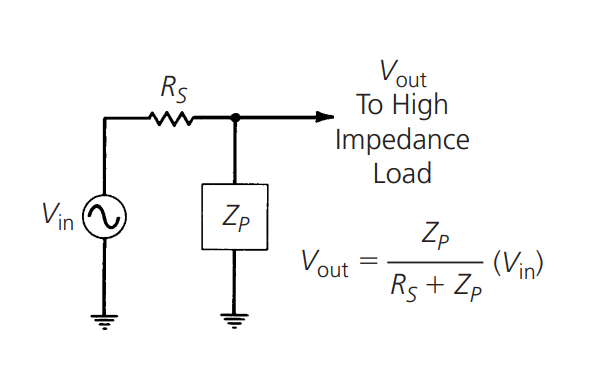$V_{out}=\frac{Z_p}{R_s+Z_p}(V_{in})$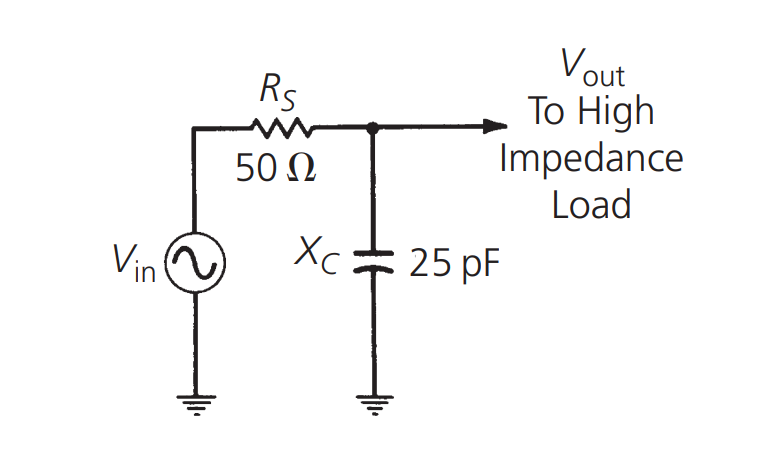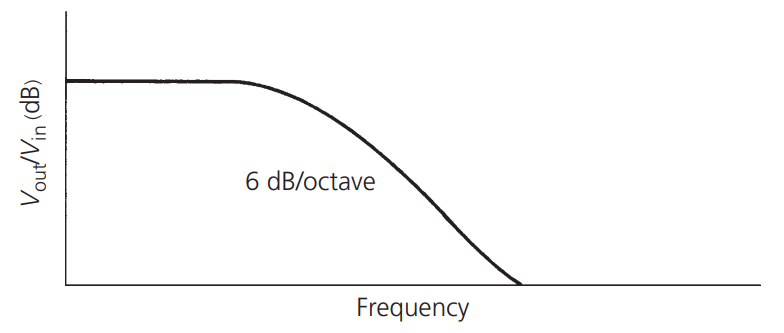$\frac{V_{out}}{V_{in}}=20\log_{10} \frac{X_C}{R_s+X_C}$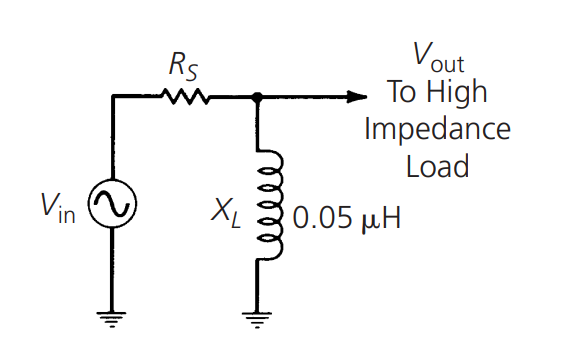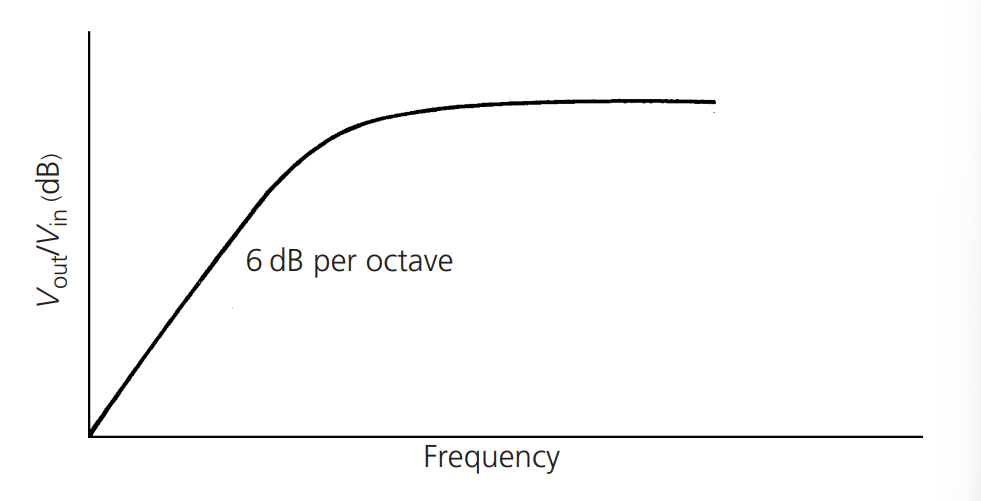$\frac{V_{out}}{V_{in}}=20\log_{10} \frac{X_L}{R_s+X_L}$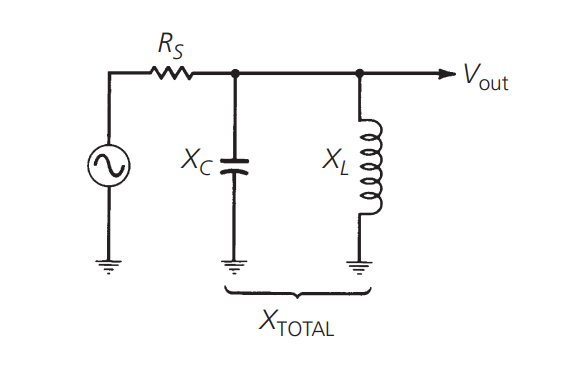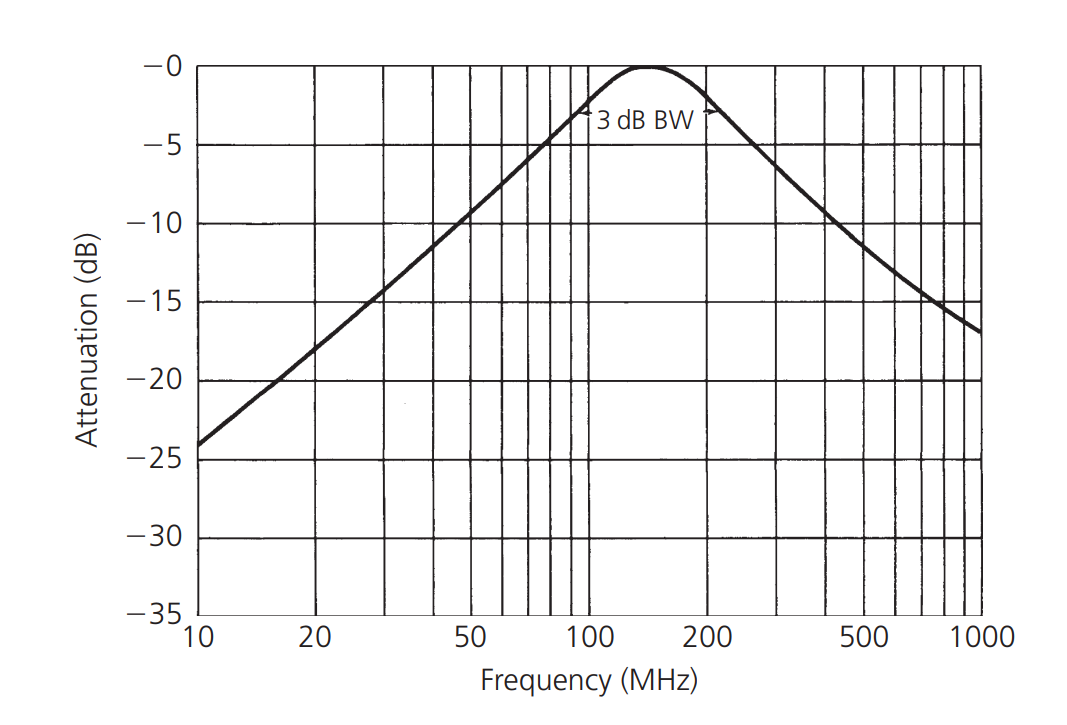$\because V_{out}=\frac{X_{total}}{R_s+X_{total}}(V_{in})$
$\because X_{total}=\frac{X_C*X_L}{X_C+X_L}$
$\because X_C=\frac{1}{j\omega C}$
$\because X_L=j\omega L$
$\therefore \frac{V_{out}}{V_{in}}=\frac{j\omega L}{(R_s-\omega^2 R_s L C)+j\omega L}$

$\frac{V_{out}}{V_{in}}= 20\log_{10} | \frac{j\omega L}{(R_s-\omega^2 R_s L C)+j\omega L} |$

RLC 滤波器可用于从环境无线电波的总频谱中选择某个窄范围的频率，作为带通滤波器使用。

## 参考与致谢

• 《RF-Circuit-Design(second-edition)_Chris-Bowick》